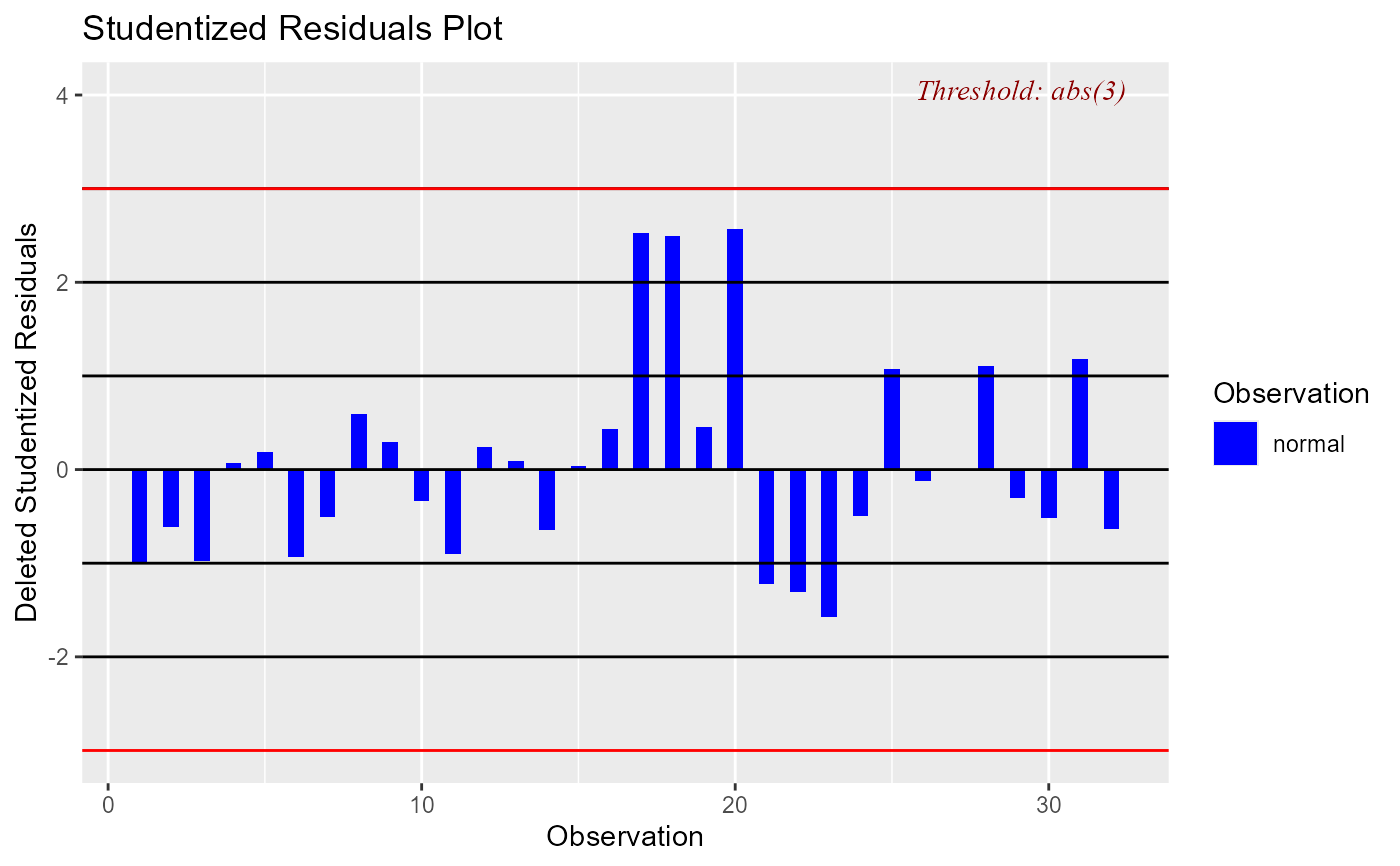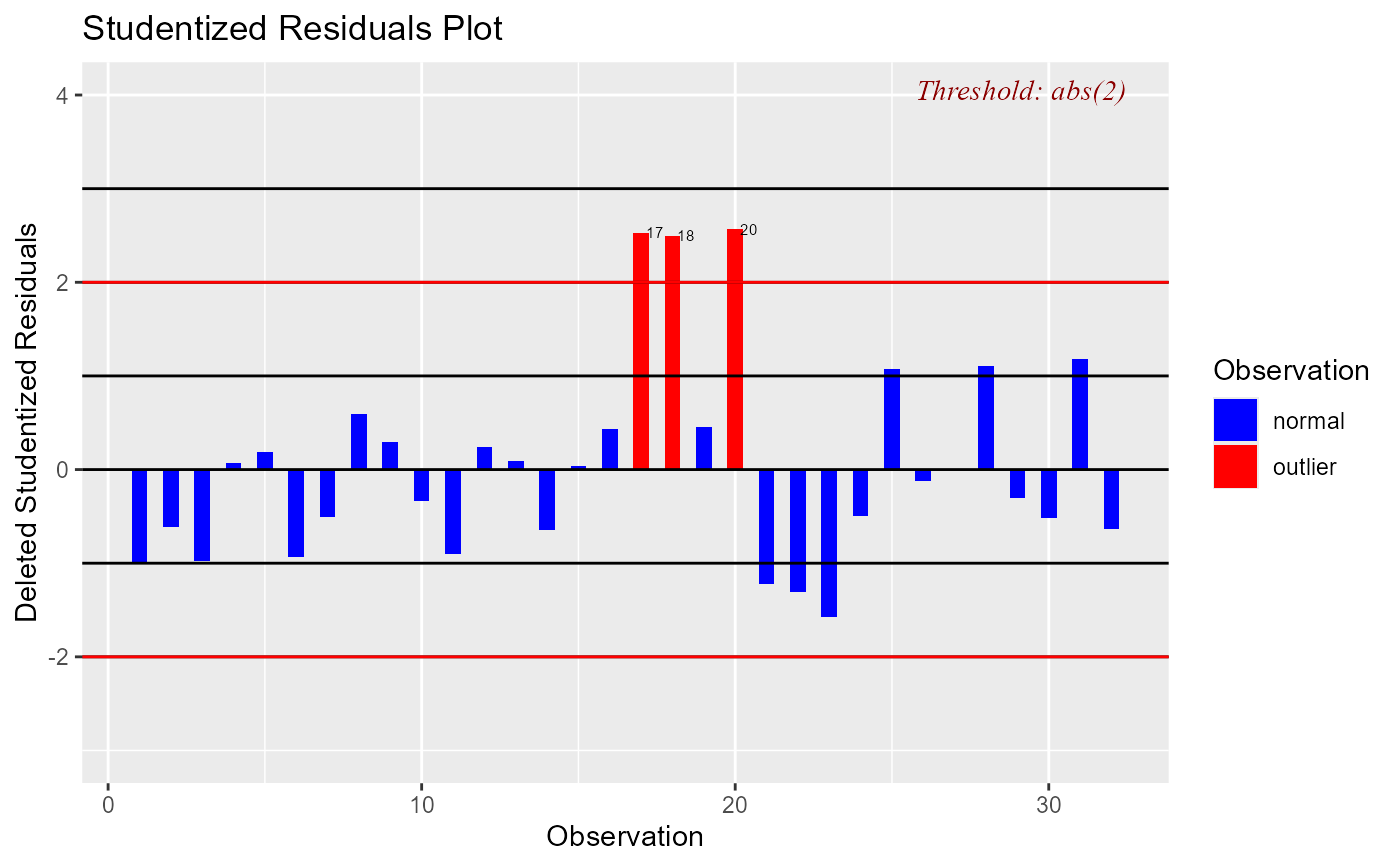Graph for identifying outliers.

ols_plot_resid_stud(model, threshold = NULL, print_plot = TRUE)

## Arguments

model An object of class lm. Threshold for detecting outliers. Default is 3. logical; if TRUE, prints the plot else returns a plot object.

## Value

ols_plot_resid_stud returns a list containing the following components:

outliers

a data.frame with observation number and studentized residuals that exceed threshold

for classifying an observation as an outlier
threshold

threshold for classifying an observation as an outlier

## Details

Studentized deleted residuals (or externally studentized residuals) is the deleted residual divided by its estimated standard deviation. Studentized residuals are going to be more effective for detecting outlying Y observations than standardized residuals. If an observation has an externally studentized residual that is larger than 3 (in absolute value) we can call it an outlier.

## Deprecated Function

ols_srsd_plot() has been deprecated. Instead use ols_plot_resid_stud().

model <- lm(mpg ~ disp + hp + wt, data = mtcars)ols_plot_resid_stud(model, threshold = 2)